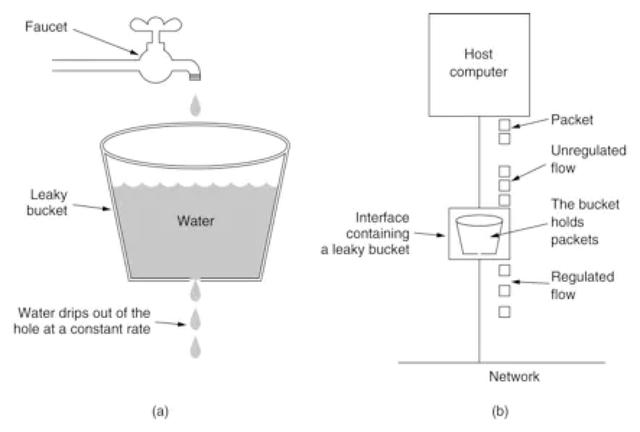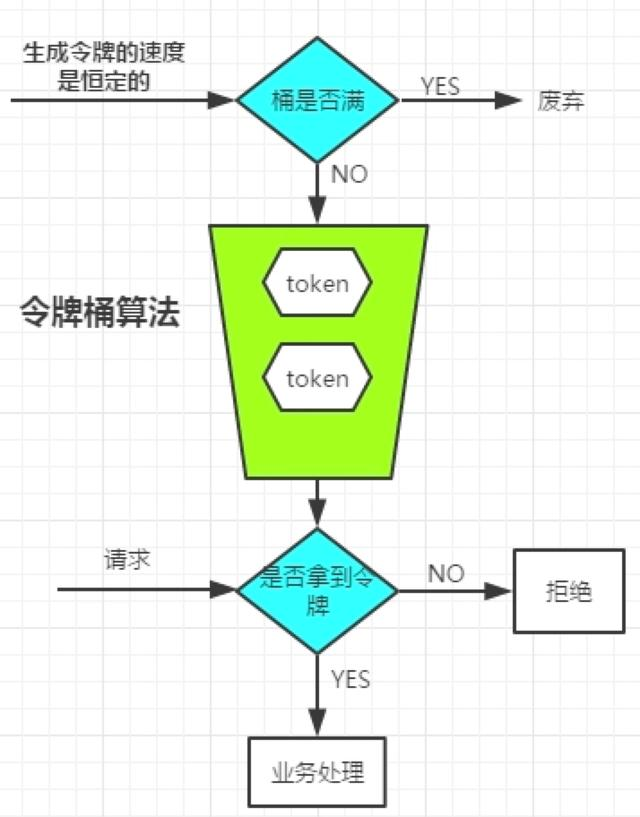技术分享 | 一文了解高并发限流算法

发布时间：2020-09-17 浏览次数：694# encoding: utf-8"""author: yangyi@youzan.comtime: 2020/9/9 10:43 PMfunc: """import timeimport multiprocessingTEST = {    # 测试 {'test1': 20} 每秒产生的令牌数量    'all': {        'test1': {'test1': 20},        'test2': {'test2': 50},        'test3': {'test3': 80},        'test4': {'test4': 100},#表示突发100个请求        'test5': {'test5': 200},#表示突发200个请求        'test6': {'test6': 20},    }}class TokenBucket(object):    # rate是令牌发放速度，capacity是桶的大小    def __init__(self, rate, capacity):        self._rate = rate        self._capacity = capacity        self._current_amount = 0        self._last_consume_time = int(time.time())    # token_amount是发送数据需要的令牌数    def consume(self, token_amount):        time.sleep(1)        # 计算从上次发送到这次发送，新发放的令牌数量        increment = (int(time.time()) - self._last_consume_time) * self._rate        # 令牌数量不能超过桶的容量        self._current_amount = min(            increment + self._current_amount, self._capacity)        # 如果没有足够的令牌，则不能发送数据        if token_amount > self._current_amount:            return False        self._last_consume_time = int(time.time())        self._current_amount -= token_amount        return Truedef job():    i = 100    while i>1:        for result in result_dict.values():            key = tuple(result.keys())            rate = tuple(result.values())            i = i-1            if i <= 0:                break            if not limiter.consume(rate):                print(key + ' 限流')            else:                print(key + ' 正常')def run():    threads = [multiprocessing.Process(target=job) for i in range(3)]    for thread in threads:        thread.start()if __name__ == '__main__':    result_dict = TEST["all"]    RATE = 30    CAPACITY = 120    limiter = TokenBucket(RATE, CAPACITY)    run()

https://github.com/titan-web/rate-limit

https://www.jianshu.com/p/c6b20845561a

https://www.simpleapples.com/2018/03/20/implementation-of-the-token-bucket-algorithm-in-python/